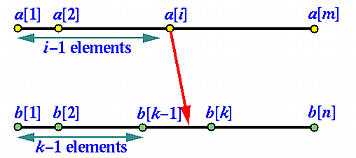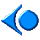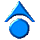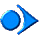Let us write a complete program for the array merging problem. Suppose we have two sorted arrays a[] of m elements and b[] of n elements. Assume further that all of these m+n elements are distinct integers.

Let us review how the merging process can be carried out with multiple threads. Take an element from array a, say a[i]. We know that it is larger than i-1 elements of a. If we can figure out how many elements of b that are smaller than a[i], we will be able to know the exact location of a[i] in the sorted array. This is illustrated in the following diagram:With a slightly modified binary search, we can easily determine the location of a[i] in array b. There are only three possibilities:

1. a[i] is less than b: In this case, a[i] is larger than i-1 elements in a and smaller than all elements in b. Therefore, a[i] should be in position i of the sorted array.
2. a[i] is larger than b[n]: In this case, a[i] is larger than i-1 elements in a and n elements in b. Therefore, a[i] should be in position i+n of the sorted array.
3. a[i] is between b[k-1] and a[k]. In this case, a[i] is larger than i-1 elements in a and k-1 elements in b. Therefore, a[i] should be in position i+k-1 of the sorted array.
After the main program reads in both arrays, it can create m+n child threads, each of which handles an element in a or in b. Each of these threads determines its position in the merged array and writes the values into the corresponding location. After this, we will have a merged array!

The first thing to do is to define a thread class for the merging threads. First, we have to decide what information a thread must carry. In the above discussion, we know that a thread that handles a[i] must have the value of a[i] and the array index i, and have the access to array b[ ]. With these information, this thread determines the location of a[i] in array b[ ]. Therefore, the class of this thread must receive the value of a[i], the index i, the array b[ ], and the number of elements in b[ ]. Hence, we have the following definition of class MergeThread in file merge.h.

 ```#include "ThreadClass.h" class MergeThread : public Thread { public: // constructor MergeThread(char whicharray, int Value, int Index, int Array[], int Result[], unsigned No_elements); private: void ThreadFunc(); // thread body int value; // a[i]: value of element index of array a[] int index; // i : index in the array a[] int *array; // another array b[] int *result; // result array char id; // identify thread name unsigned no_of_elements; // number of elements of array B[] }; ``` Click here to download this file (merge.h)

The above is quite clear and the missing parts are a constructor and the execution code of this thread, which should go into method ThreadFunc(). In the constructor below, for the purpose of easy identification, we give the thread a name that is related to index value index it receives. It is not difficult to modify the arguments so that the thread name can indicate if it is handling element a[i] or element b[j]. The following shows a possible solution for merging thread, which is based on a modified binary search. In this code segment, we try to determine if the value of value is in one of the three regions: (1) lower to middle, (2) middle to middle+1, or (3) middle+1 to upper. If case (3) holds, we are done; otherwise, we reduce the bound just like the binary search does. Once the position is calculated, the value held by this thread, value, is stored into the correct position of the global array result[ ]. Let us save this implementation to file merge.cpp

 ```#include #include "merge.h" MergeThread::MergeThread(char whicharray, int Value, int Index, int Array[], int Result[], unsigned No_elements) :id(whicharray), value(Value), index(Index), result(Result), array(Array), no_of_elements(No_elements) { ThreadName.seekp(0, ios::beg); ThreadName << "Merging" << id << '-' << index << '\0'; } void MergeThread::ThreadFunc() { Thread::ThreadFunc(); int lower, upper, middle; if (this->value < array) result[index] = this->value; else if (this->value > array[no_of_elements - 1]) result[no_of_elements + index] = this->value; else { lower = 0; upper = no_of_elements - 1; while (upper > lower) { middle = (upper + lower)/2; if(value > array[middle] && value < array[middle + 1]) break; else if(value < array[middle]) upper = middle; else if(value > array[middle]) lower = middle + 1; } result[index + middle + 1] = this->value; } // thread exit Exit(); } ``` Click here to download this file (merge.cpp)

Let us consider the main program. In fact, it is very simple. The following is a simplified version. First, array a[] of m elements and array b[] of n elements are read in. Then, create m threads to determine the position of a[i] for 0 <= i < m, and n threads to determine the position of b[i] for 0 <= i < n. After this is done, the main thread waits until all of its m+n child threads complete. Finally, it prints out the merged array result[].

 ```#include #include "merge.h" #define MAX_SIZE 50 int main(int argc, char *argv[]) { int a[MAX_SIZE], // sorted array a b[MAX_SIZE], // sorted array b result[MAX_SIZE * 2]; // result array int m; // no. of elements of a[] int n; // no. of elements of b[] int i; MergeThread *Group_A[MAX_SIZE]; // find the position of a[i] in b[] MergeThread *Group_B[MAX_SIZE]; // find the position of b[j] in a[] cout << "Please input size for array a: " << endl; cin >> m; cout << "Please input the elements of array a: " << endl; for (i = 0; i < m; i++) cin >> a[i]; cout << "Please input size for array b: " << endl; cin >> n; cout << "Please input the elements of array b: " << endl; for (i = 0; i < n; i++) cin >> b[i]; // print out array before merging cout << "Before merging: array a is:" << endl; for (i = 0; i < m; i++) cout << a[i] << " "; cout << endl; cout << "Before merging: array b is:" << endl; for (i = 0; i < n; i++) cout << b[i] << " "; cout << endl; for (i = 0; i < m; i++) { // fire up merging threads for a[i]'s Group_A[i] = new MergeThread('A', a[i], i, b, result, n); Group_A[i]->Begin(); } for (i = 0; i < n; i++) { // fire up merging threads for b[i]'s Group_B[i] = new MergeThread('B', b[i], i, a, result, m); Group_B[i]->Begin(); } // wait for all the child threads for (i = 0; i < m; i++) Group_A[i]->Join(); for (i = 0; i < n; i++) Group_B[i]->Join(); // print out the merged array cout << "After merging, the result array is:" << endl; for (i = 0; i < m + n; i++) cout << result[i] << " "; cout << endl; Exit(); return 0; } ``` Click here to download this file (merge-main.cpp)